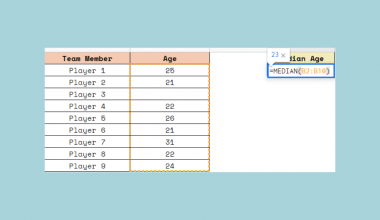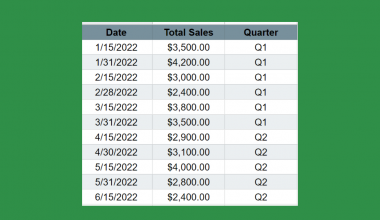# How to Use TODAY Function in Google Sheets

The TODAY function in Google Sheets is useful to return the current date.

We can use the `TODAY` function on its own as a standalone formula to return the current date. However, it’s more useful to build it in dynamic formulas that need the current date as an input variable.

Let’s take an example.

We want to calculate how many days have passed since a specific date and we always want to keep this information updated.

So how do we do that?

It’s pretty simple. The `TODAY` function doesn’t need any parameters to return the current date automatically every day. We can use it in any calculations that need this information.

Let’s dive right into some examples where we will deal with actual values and see how we can write our own TODAY function in Google Sheets.

## The Anatomy of the TODAY Function

The syntax of a function tells us how we should write and use the function. In which case, the syntax of the `TODAY` function is as follows:

`=TODAY()`

Let’s understand what this terminology means:

• `=` the equal sign is how we start all functions in Google Sheets.
• `TODAY` is our function. Unlike other functions, the `TODAY` function doesn’t take any input variables.

### ⚠️ Now A Few Notes to Make Your TODAY Function Work Perfectly

1. You need to write the brackets even though there shouldn’t be anything in them. The brackets should be empty; otherwise, the function gives an error.
2. The `TODAY` function is one of the volatile functions of Google Sheets. This means that it causes recalculation on every sheet change. This can have an impact on sheet performance.
3. This also means that the `TODAY` function will always represent the current date the last time the spreadsheet was recalculated, and it doesn’t remain at the date when the function was first entered.
4. The `TODAY` formula returns the current date as the local date settings on the user’s computer. It obtains this information by reading the computer’s clock.
5. `TODAY` provides the current date with no time component. You can create a date with the current time by using the similar `NOW` function.

## A Real Example of Using TODAY Function

Let’s look at some examples of how to use the TODAY function in Google Sheets.

### Use TODAY Function as a Standalone Function

This picture shows how can we use the `TODAY` function on its own.As simple as it can get, the function outputs the current date. Of course, it was the current date when the picture was taken 🙂

We simply clicked on an empty cell and typed the following function:

`=TODAY()`

The most important thing to remember is that this value is updated every time when the document recalculates. As we have said, this is called a volatile function.

This means that it doesn’t remain at the date when we first used the formula, but it changes dynamically to the current date whenever we perform any activity in the sheet, including when we open it.

For example, the `TODAY` formula shows ‘5/8/2020‘ now. But if we open the sheet two days later, the result of the formula will change to ‘5/10/2020‘.

Nevertheless, there may be cases when you don’t want this behaviour but want the date to always remain the same. Then you need to add the date manually without the `TODAY` function. Alternatively, you can use a keyboard shortcut which is ‘Ctrl + ;’  (semi-colon) for a static date.

### Use TODAY Function as Part of Another Formula

Let’s see how can we use the `TODAY` function as an input variable in other functions.The above image shows how to use the TODAY function in Google Sheets to calculate how many days have passed since a specific date.

We have some dates in the range from A4 to A11. Firstly, we calculated the number of days since the first date in cell A4.

The following formula will do this:

`=TODAY()-A4`

Here’s what this example does:

• Firstly, we selected cell B4. This is where we wrote our first formula.
• We used the `TODAY` function to get the current date.
• After that, we subtracted the content of cell A4. By doing this, we calculated the difference between the current date and the specific date written in cell A4.
• As a result, we got 6335. This is the number of days that passed between 1/3/2003 and the current date at the time of creating this example which is 5/8/2020.
• We dragged down the formula to apply it to the other dates and show the results for every date in column A. Apart from dragging, we presented various ways to copy a formula down an entire column in Google Sheets. Check out our article about it!

The `TODAY` function in the cells makes these results update automatically without manually recalculating them. So anytime we open the sheet, we see the updated date difference between the dates in column A and the current date.

It’s simple, right?

You can make a copy of the spreadsheet using the link I have attached below and have a go at it yourself:

## How to Use TODAY Function in Google Sheets

Let’s see how to write your own TODAY function in Google Sheets step-by-step to use it in the above example.

1. To begin, click on the cell where you want your results to show. For the this guide, I will be choosing B4, where I will write my first formula.1. Next, type the equal sign ‘=’ to begin the function and then followed by the name of the function which is ‘`today`‘ (or ‘`TODAY`‘, whichever works).1. You should now see that the auto-suggest box will pop-up with the name and explanation of the `TODAY` function. Click on it, and Google Sheet will put the ‘(‘ opening brackets for you.1. After that, you simply need to close these brackets. The `TODAY` function doesn’t take any variables, so you shouldn’t put anything in it. You only need the empty ‘()‘ brackets after the name of the function.1. Now you can write your calculation in which you wish to use the current date. For example, I used it to calculate the number of days between the current date and some specific dates. To do this, I subtracted the content of cell A4.1. Finally, I applied the formula to my whole data set, so down until cell B11. As a result, I got a dynamically changing list that returns the current result every day.That’s it, well done! You can now use the `TODAY` function together with the various other Google Sheets formulas to create even more useful formulas. 🙂Our goal this year is to create lots of rich, bite-sized tutorials for Google Sheets users like you. If you liked this one, you'll love what we are working on! Readers receive ✨ early access ✨ to new content.

##### You May Also Like## How to Add a Vertical Line to a Line Chart in Google Sheets

This guide will explain how to add a vertical line to a line chart in Google Sheets. Users…## How to Use POISSON.DIST Function in Google Sheets

The POISSON.DIST function in Google Sheets is useful when you want to return the value of the Poisson…## How to Overflow Text in Google Sheets

In this article, you will learn how to unwrap a text in Google Sheets using the Overflow wrapping…## How to Use GROWTH Function in Google Sheets

The GROWTH function in Excel is useful in predicting future values for your business. This function helps predict…